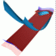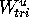Abstract and Contents
Next: Animations
Previous: References

# List of all figures

 Figure 1 Possible dynamics on two-dimensional stable and unstable manifolds. Figure 2 The influence of the dynamics when iterating points. Figure 3 The linearized stable and unstable manifolds of a circle of saddle-type. Figure 4 The algorithm GLOBALIZE in pseudo-code. Figure 5 The procedure ADDCIRCLE in pseudo-code. Figure 6 Linear foliation near the invariant circle H. Figure 7 The map acting on triangles of. Figure 8 Initial mesh near a hyperbolic fixed point. Figure 9 Mesh adaptation by adding extra leaves of the foliation. Figure 10 Two different viewpoints of the stable manifold of the 3D-fattened Arnol'd family. Figure 11 The unstable manifold of the Quasiperiodically forced Hénon map. Figure 12 Heteroclinic tangle of two-dimensional stable and unstable manifolds of the Quasiperiodically forced Hénon map. Figure 13 The unstable manifold of the origin of the example of a hyperbolic fixed point. Figure 14 Comparing the condition of the mesh when leaves are added and when not. Figure 15 The stable manifold of the Lorenz system computed by [Worfolk 1997]. Figure 16 The stable manifold of the Lorenz system computed with our algorith Figure 17 Stable and unstable manifolds of the normal form of the Hopf-Hopf bifurcation. Figure 18 A situation where the Foliation Condition is not satisfied.

Next: Animations
Previous: ReferencesAbstract and Contents

Written by: Bernd Krauskopf & Hinke Osinga
Created: May 27 1997 --- Last modified: Tue Jun 10 13:22:16 1997